# Vertical tangent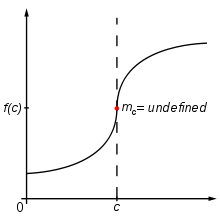Vertical tangent on the function ƒ(x) at x=c.

In mathematics, particularly calculus, a vertical tangent is tangent line that is vertical. Because a vertical line has infinite slope, a function whose graph has a vertical tangent is not differentiable at the point of tangency.

## Limit definition

A function ƒ has a vertical tangent at x = a if the difference quotient used to define the derivative has infinite limit: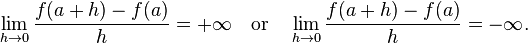$\lim_{h\to 0}\frac{f(a+h) - f(a)}{h} = {+\infty}\quad\text{or}\quad\lim_{h\to 0}\frac{f(a+h) - f(a)}{h} = {-\infty}.$

The first case corresponds to an upward-sloping vertical tangent, and the second case to a downward-sloping vertical tangent. Informally speaking, the graph of ƒ has a vertical tangent at x = a if the derivative of ƒ at a is either positive or negative infinity.

For a continuous function, it is often possible to detect a vertical tangent by taking the limit of the derivative. If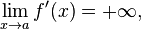$\lim_{x\to a} f'(x) = {+\infty}\text{,}$

then ƒ must have an upward-sloping vertical tangent at x = a. Similarly, if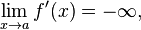$\lim_{x\to a} f'(x) = {-\infty}\text{,}$

then ƒ must have a downward-sloping vertical tangent at x = a. In these situations, the vertical tangent to ƒ appears as a vertical asymptote on the graph of the derivative.

## Vertical cusps

Closely related to vertical tangents are vertical cusps. This occurs when the one-sided derivatives are both infinite, but one is positive and the other is negative. For example, if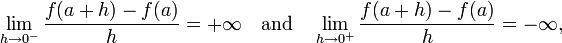$\lim_{h \to 0^-}\frac{f(a+h) - f(a)}{h} = {+\infty}\quad\text{and}\quad \lim_{h\to 0^+}\frac{f(a+h) - f(a)}{h} = {-\infty}\text{,}$

then the graph of ƒ will have a vertical cusp that slopes up on the left side and down on the right side.

As with vertical tangents, vertical cusps can sometimes be detected for a continuous function by examining the limit of the derivative. For example, if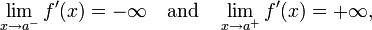$\lim_{x \to a^-} f'(x) = {-\infty} \quad \text{and} \quad \lim_{x \to a^+} f'(x) = {+\infty}\text{,}$

then the graph of ƒ will have a vertical cusp that slopes down on the left side and up on the right side. This corresponds to a vertical asymptote on the graph of the derivative that goes to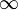$\infty$ on the left and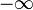$-\infty$ on the right.

## Example

The function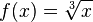$f(x) = \sqrt{x}$

has a vertical tangent at x = 0, since it is continuous and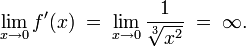$\lim_{x\to 0} f'(x) \;=\; \lim_{x\to 0} \frac{1}{\sqrt{x^2}} \;=\; \infty.$

Similarly, the function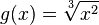$g(x) = \sqrt{x^2}$

has a vertical cusp at x = 0, since it is continuous,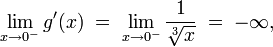$\lim_{x\to 0^-} g'(x) \;=\; \lim_{x\to 0^-} \frac{1}{\sqrt{x}} \;=\; {-\infty}\text{,}$

and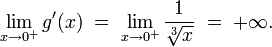$\lim_{x\to 0^+} g'(x) \;=\; \lim_{x\to 0^+} \frac{1}{\sqrt{x}} \;=\; {+\infty}\text{.}$

## References

Vertical Tangents and Cusps. Retrieved May 12, 2006.